IMO Shortlist 2011 problem N1

Kvaliteta:
Avg: 0,0
Težina:
Avg: 6,0
For any integer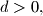$d > 0,$ let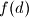$f(d)$ be the smallest possible integer that has exactly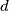$d$ positive divisors (so for example we have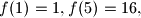$f(1)=1, f(5)=16,$ and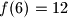$f(6)=12$). Prove that for every integer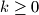$k \geq 0$ the number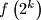$f\left(2^k\right)$ divides$f\left(2^{k+1}\right).$

Proposed by Suhaimi Ramly, Malaysia
Izvor: Međunarodna matematička olimpijada, shortlist 2011